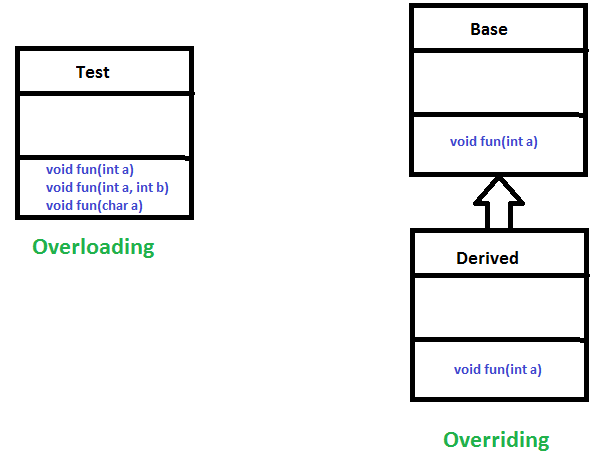Related Articles

# Polymorphism in Java

• Difficulty Level : Easy
• Last Updated : 01 Dec, 2020

The word polymorphism means having many forms. In simple words, we can define polymorphism as the ability of a message to be displayed in more than one form.

Real life example of polymorphism: A person at the same time can have different characteristic. Like a man at the same time is a father, a husband, an employee. So the same person posses different behavior in different situations. This is called polymorphism.

Polymorphism is considered one of the important features of Object-Oriented Programming. Polymorphism allows us to perform a single action in different ways. In other words, polymorphism allows you to define one interface and have multiple implementations. The word “poly” means many and “morphs” means forms, So it means many forms.

In Java polymorphism is mainly divided into two types:

• Compile time Polymorphism
• Runtime PolymorphismMethod Overloading: When there are multiple functions with same name but different parameters then these functions are said to be overloaded. Functions can be overloaded by change in number of arguments or/and change in type of arguments.

Example: By using different types of arguments

 `// Java program for Method overloading`` ` `class` `MultiplyFun {`` ` `    ``// Method with 2 parameter``    ``static` `int` `Multiply(``int` `a, ``int` `b)``    ``{``        ``return` `a * b;``    ``}`` ` `    ``// Method with the same name but 2 double parameter``    ``static` `double` `Multiply(``double` `a, ``double` `b)``    ``{``        ``return` `a * b;``    ``}``}`` ` `class` `Main {``    ``public` `static` `void` `main(String[] args)``    ``{`` ` `        ``System.out.println(MultiplyFun.Multiply(``2``, ``4``));`` ` `        ``System.out.println(MultiplyFun.Multiply(``5.5``, ``6.3``));``    ``}``}`
Output:
```8
34.65
```

Example 2: By using different numbers of arguments

 `// Java program for Method overloading`` ` `class` `MultiplyFun {`` ` `    ``// Method with 2 parameter``    ``static` `int` `Multiply(``int` `a, ``int` `b)``    ``{``        ``return` `a * b;``    ``}`` ` `    ``// Method with the same name but 3 parameter``    ``static` `int` `Multiply(``int` `a, ``int` `b, ``int` `c)``    ``{``        ``return` `a * b * c;``    ``}``}`` ` `class` `Main {``    ``public` `static` `void` `main(String[] args)``    ``{``        ``System.out.println(MultiplyFun.Multiply(``2``, ``4``));`` ` `        ``System.out.println(MultiplyFun.Multiply(``2``, ``7``, ``3``));``    ``}``}`
Output:
```8
42
```

2. Runtime polymorphism: It is also known as Dynamic Method Dispatch. It is a process in which a function call to the overridden method is resolved at Runtime. This type of polymorphism is achieved by Method Overriding.

Method overriding, on the other hand, occurs when a derived class has a definition for one of the member functions of the base class. That base function is said to be overridden.

Example:

 `// Java program for Method overriding`` ` `class` `Parent {`` ` `    ``void` `Print()``    ``{``        ``System.out.println(``"parent class"``);``    ``}``}`` ` `class` `subclass1 ``extends` `Parent {`` ` `    ``void` `Print()``    ``{``        ``System.out.println(``"subclass1"``);``    ``}``}`` ` `class` `subclass2 ``extends` `Parent {`` ` `    ``void` `Print()``    ``{``        ``System.out.println(``"subclass2"``);``    ``}``}`` ` `class` `TestPolymorphism3 {``    ``public` `static` `void` `main(String[] args)``    ``{`` ` `        ``Parent a;`` ` `        ``a = ``new` `subclass1();``        ``a.Print();`` ` `        ``a = ``new` `subclass2();``        ``a.Print();``    ``}``}`
Output:
```subclass1
subclass2
```

Attention reader! Don’t stop learning now. Get hold of all the important Java Foundation and Collections concepts with the Fundamentals of Java and Java Collections Course at a student-friendly price and become industry ready. To complete your preparation from learning a language to DS Algo and many more,  please refer Complete Interview Preparation Course.

My Personal Notes arrow_drop_up